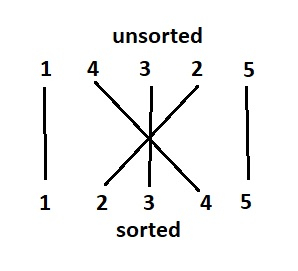# Counting cross lines in an array in C++

We are given with an array of distinct elements that are unsorted. The goal is to find the cross lines after the array is sorted. Cross lines are counted as shown below −

• Arr[]={ 1,2,4,3,5 } There are 3 cross lines as shown below• Arr[]= { 1,2,3,4,5 }. There are no cross lines as the array is already sorted.

We will count the cross lines using insertion sort in which an element from right is added to sorted elements on its left. Each time the element is added in the sorted part, increment count as it moves to its correct position. It will pass by crossing all elements that are greater.

Let’s understand with examples.

Input − arr[]= {4,3,1,2 }

Output− Count of cross lines in array − 5

Explanation − Line 4-4 and 3-3 crosses lines 1-1 and 2-2. Total 4 cross lines.

Both 4-4 and 3-3 cross each other once. Total 4+1=5 cross lines.

Input − arr[]= { 0,1,5,3 }

Output − Count of cross lines in array − 1

Explanation − Both 5-5 and 3-3 cross each other once. Total 1 cross line.

## Approach used in the below program is as follows

• We take an integer array arr[] initialized with distinct numbers.

• Function insertionSort(int arr[], int n) takes an array and its length as input and after sorting it returns the count of cross lines as result.

• Take initial no of cross lines as 0. Count variable is used.

• First element is already sorted, so starting from the second element till end (i=1 to i<n), take each element in the item. (item=arr[i]) and j=i-1.

• Now shift all elements right if they are > item and j>0. For each shift increment count as these all are crossed by item.

• At the end of the while loop place item at its correct position that is arr[j+1].

• Do this for all elements and count elements they cross.

• Increment value of count as no. of cross lines possible.

## Example

Live Demo

#include <bits/stdc++.h>
using namespace std;
int insertionSort(int arr[], int n){
int count=0;
int item;
int j;
for (int i = 1; i < n; i++){
item = arr[i];
j = i - 1;
//insert element at correct position in sorted part, no. of elements it passes
//from right till correct position is count of cross lines.
while (j >= 0 && arr[j] > item){
arr[j + 1] = arr[j];
j = j - 1;
count++;
}
arr[j + 1] = item;
}
return count;
}
int main(){
int arr[] = { 4,5,3,1,2};
int n = 5;
cout<<"Number of cross lines: "<<insertionSort(arr, n);
return 0;
}

## Output

If we run the above code it will generate the following output −

Number of cross lines: 8